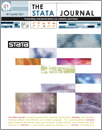Home  >>  Archives  >>  Volume 9 Number 2  >>  st0162

#### The Stata JournalVolume 9 Number 2: pp. 175-196

Subscribe to the Stata Journal## Methods for estimating adjusted risk ratios

 Peter Cummings Department of Epidemiology School of Public Health and Harborview Injury Prevention and Research Center University of Washington Seattle, WA peterc@u.washington.edu
Abstract.  The risk ratio can be a useful statistic for summarizing the results of cross-sectional, cohort, and randomized trial studies. I discuss several methods for estimating adjusted risk ratios and show how they can be executed in Stata, including 1) Mantel–Haenszel and inverse-variance stratified methods; 2) generalized linear regression with a log link and binomial distribution; 3) generalized linear regression with a log link, normal distribution, and robust variance estimator; 4) Poisson regression with a robust variance estimator; 5) Cox proportional hazards regression with a robust variance estimator; 6) standardized risk ratios from logistic, probit, complementary log-log, and log-log regression; and 7) a substitution method. Advantages and drawbacks are noted for some methods.

View all articles by this author: Peter Cummings

View all articles with these keywords: risk ratio, odds ratio